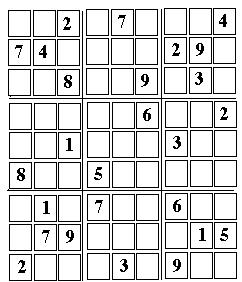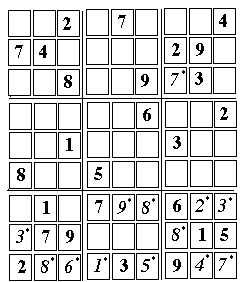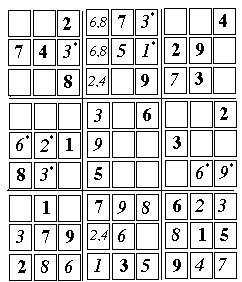Site Map

## Sudoku: step-by-step solution of a harder puzzle# Solving a Harder Sudoku Puzzle

### What you see and what you get

This is a step by step solution of a random Sudoku "devilish" puzzle, intended to demonstrate additional methods of solution. It is a follow-up to a web page analyzing a simpler puzzle--simpler, though not too easy, either. If you have not done so, it might be a good idea to start there.

As before, follow the steps listed below provide an idea of how the solution is derived. There may exist other approaches--but here are the methods I used, and they worked. The array used is pictured above; it is suggested you bring it up on the screen (in larger format) by clicking here, print it and then use a pencil to fill in the empty squares, in the order of the steps outlined below (you may add in the corner of each cell filled its number in the sequence of steps, to help retracing your route). As before, some intermediate stages of the solution will be shown, with added numbers marked with *.

### (the lines below are repeated from the earlier puzzle)

Sudoku comes from Japan (it originated in the US in 1979, but became popular in Japan after 1984--see article in "Scientific American" cited at the bottom) and is based on what mathematicians call "Latin Squares"--square arrays of numbers in which every number appears exactly once in any row and in any column (different letters, colors or symbols may be used in place of the numbers).

A Sudoku puzzle contains 9 rows of 9 numbers, each containing (1, 2, 3 ... 9) in a different order, and the same holds for the columns. In addition, however, each Sudoku array can be divided into 9 smaller 3-by-3 squares, each of which which also must contain all the numbers (1,2,3....9). In fact many such arrays exist.

The challenge of the puzzle is--given just some of the numbers of a specific array, fill in the missing ones, using only logical deduction based on the above requirements.

The puzzle consists of a square array of 81 cells, which can be divided into 9 columns, (counted from the left), each containing 9 cells. In each column, cells are numbered from the bottom.

Or else, it can be divided into 9 rows (counted from the bottom), each containing 9 cells. In each row, cells are numbered from the left.

A notation like 6 (3,2) will mean the number "6" in cell 2 of column 3

Extra division lines (or darker ones) divide the array into 9 boxes, each with 3 rows of 3 cells each. To define the positions of boxes, it is easiest to classify them by stacks and tiers:

Three boxes in a vertical columns will be called a stack
(distinguishing left, right or middle stacks)
Three boxes in a horizontal row will be called a tier
(distinguishing bottom, top or middle tiers)

The requirement of the puzzle:
Every column, row and box must contain all the nine numbers 1,2,3 ... 9.
No number may be repeated, none can be left out.

### Added Comment on Unresolved Pairs

Additional notes on solving the puzzle can be found with the preceding puzzle. The hints there include "solution rule 2" or SR2:
The same number cannot appear twice in any row, column or box. Therefore, we look for cells whose row, column and box contain between them a wide variety of numbers.
If that variety includes all numbers except for one, that remaining number is the only choice remaining.
In the puzzle used earlier as an example, the upper right corner cell was a good candidate for this approach. Its row contained (8, 5, 1, 4, 3), its column (9, 4, 2, 6, 8) and its box (in addition to the cells mentioned above), (8, 7). Thus all numbers but "9" were accounted for, showing that "9" belonged in the cell: any other number in that cell would have forced a duplication.

Here this rule is not sufficient, and we may need use cells where, on applying this method, all numbers but two are eliminated.

By itself, this information is not particularly useful--knowing, for instance, that the only numbers which can appear in that cell would be 2 or 7. However, if we test another cell in the same box and also find that the number in it must be either 2 or 7, then we have an unresolved pair. These two cells must contain 7 and 2: we do not know in which order--and it may take a while before we do--but in filling other cells in the same box, we may safely assume that the number needed is not either 7 or 2.

Instead of "box" we may equally write "row" or "column". In particular, if all cells except for two in a row or column are filled, the two cells left are also an unresolved pair. That is no help in filling other cells in that row or column--they are already full!--but if these two cells also belong to the same box, the information may help fill other cells there.
In principle, it is possible to need to identify unresolved groups of more than two cells. In the case below, two unresolved pairs together help fill another cell in the same box, so that we are really using a group of 4 unresolved cells, arranged in two pairs. Larger unresolved groups make the puzzle more difficult. With a pair, once one of the cells is filled, the other one is also solved, but with larger groups that is no longer true.

Numbers entered as part of the solution will be introduced in the order in which they are derived, numbered in parentheses. Copy the puzzle here, print it and enter numbers as they are derived. Unresolved pairs may be written in tiny numerals--and if you use a pencil, you can erase ambiguous entries when they can be replaced by final ones.The first 13 cells are relatively easy--but can't be skipped, they will be needed later.

     2 (8,3)
Only one choice (OOC) exists here
     3 (9,3)
Only one choice (OOC) exists.
     3 (1,2)
OOC here too. Couldn't have got here without the preceding step!
     8 (2,1)
OOC here too
     9 (5,3)
OOC here too (in the rows)
     6 (3,1)
OOC here too. We are trying to fill the bottom left box.
     8 (6, 3)
OC for an 8 in the 3rd row8 (7, 2)
OC for an 8 in that row. We are on our way to filling the bottom right box.
     4 (8,1)
OOC a 4 in that box.
     7 (9,1)
Last cell left in the box
     5 (6,1)
OOC
     7 (7,7)
OOC
     1 (4,1)
OOC... but here the easy choices have run out.
--------------------------------
Now choices become limited. Using SR2--in filling an empty call, excluding all numbers of the same row, column or box--may leave one not with one choice but two. In the middle box of the top tier

(6, 9) can be 1 or 3
(6, 8) can be 1 or 3 , too.
Therefore, the numbers (1, 3) in that box are an "unresolved pair"--they occupy those cells, but in an unknown order.
Similarly, in the same box, since (1,3) are already in one of the above cells

(4, 9) can be 6 or 8 only
(4, 8) can be 6 or 8 only, too, by a similar argument.
Therefore, (6, 8) in that box are another unresolved pair (UP).5 (5, 8)
With (1,3,6, 8) excluded by unresolved pairs in the box, and (2, 4, 7, 9) disqualified by sharing the same row or column, this is the only choice.

     6 (5, 2)
By the above argument, column 4 has a "6" (even though we don't know in which of two cells it is). Therefore "6" must occupy this cell--each adjacent column already includes that number.

The top box and the bottom box in the middle stack each have two empty cells, in the same row. Taking into account cells with known contents (including unresolved pairs in the top box), these cells must contain either 2 or 4. Two of the cells on top and bottom share the same column, and therefore their numbers must differ. They too, are an unresolved pair. (Each also forms a separate UP with the empty cell to its right, but that is not used right now). In the drawing above, UPs are denoted by two numbers sharing the same cell

Even if the order of 2 and 4 in the above pair is not known, the remaining two cells in the 4th column must contain 3 and 9. There is already a 3 at (7, 5), so
     3 (4, 6),    and
     9 (4, 5)
----------------------------
     3 (3, 8)
Next, using SR2 gives . Filling this cell resolves the order of one unresolved pair:
     3 (6, 9)
     1 (6, 8)9 (9, 4)
The "9" in  leaves no other choice.

     3 (2, 4)
No other place in this box allows a "3"

     2 (2, 5)
There must exist a "2" in this box and column.
Also, the middle box of the first stack has only one place open for the "6":
     6 (1, 5)
from which
     6 (8, 4)